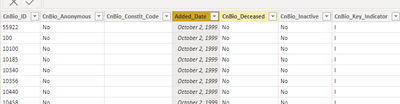cancel
Showing results for
Did you mean:Frequent Visitor

## Count based on condition - A table of multiple values was supplied where a single value was expected

Hello,

I'm trygin to count the number of rows that meet the criteria specified in Filter however the results is "A table of multiple values was supplied where a single value was expected"

AcquisitionFY22 = CALCULATE(
COUNTROWS(Donors),
FILTER(Donors,
Table snip:1 ACCEPTED SOLUTIONSuper User

FILTER needs a boolean expression as its second argument, try this instead

``````AcquisitionFY22 =
CALCULATE (
COUNTROWS ( Donors ),
DATESBETWEEN (
DATE ( 2021, 04, 01 ),
DATE ( 2022, 03, 31 )
)
)
``````
2 REPLIES 2Frequent Visitor

Thanks !!!Super User

FILTER needs a boolean expression as its second argument, try this instead

``````AcquisitionFY22 =
CALCULATE (
COUNTROWS ( Donors ),
DATESBETWEEN (
DATE ( 2021, 04, 01 ),
DATE ( 2022, 03, 31 )
)
)
``````